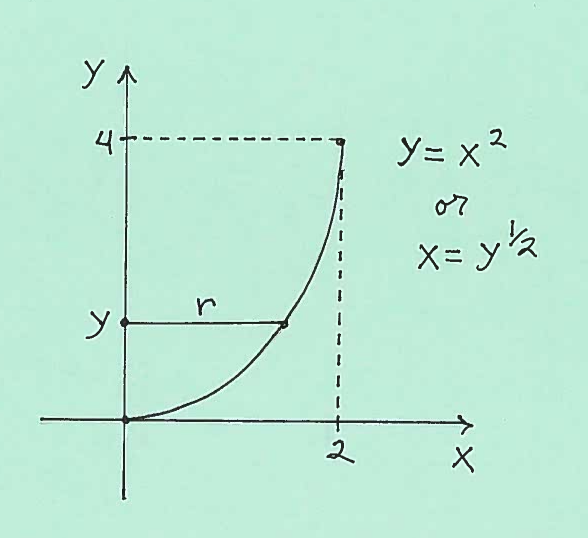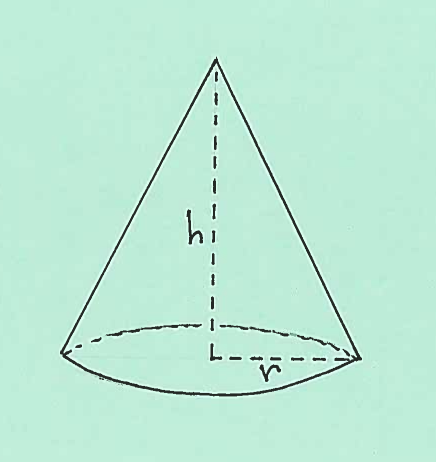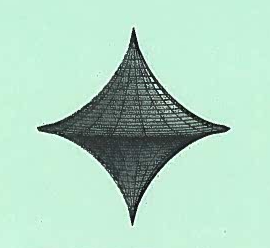### COMPUTING THE AREA OF A SURFACE OF REVOLUTION

The following problems will use the Shell Method to find the Volume of a Solid of Revolution. We start with a region $R$ in the $xy$-plane, which we "spin" around the $y$-axis to create a Solid of Revolution. Imagine the solid composed of thin concentric "shells" or cylinders, somewhat like layers of an onion, with centers of the shells being the $y$-axis. This process is called the Shell Method. The details of the Shell Method are posted below.When doing problems we will first sketch a graph of the function on a specific interval. If we are revolving the graph of $y=f(x)$ about the $x$-axis, we will mark the radius $r=f(x)$ at $x$ on the $x$-axis for $a \le x \le b$. If we are revolving the graph of $x=g(y)$ about the $y$-axis, we will mark the radius $r=g(y)$ at $y$ on the $x$-axis for $c \le x \le d$. Then the total Surface Area of the Surface of Revolution is either $$Surface \ Area = 2 \pi \int_{a}^{b} (radius) \sqrt{ 1 + \Big({dy \over dx}\Big)^2 } \ dx = 2 \pi \int_{a}^{b} (f(x)) \sqrt{ 1 + \Big({dy \over dx}\Big)^2 } \ dx$$ or $$Surface \ Area = 2 \pi \int_{c}^{d} (radius) \sqrt{ 1 + \Big({dx \over dy}\Big)^2 } \ dy = 2 \pi \int_{c}^{d} (g(y)) \sqrt{ 1 + \Big({dx \over dy}\Big)^2 } \ dy$$

EXAMPLE 1: Consider the graph of $y=x^3$ on the interval $0 \le x \le 2$. Compute the Area of the Surface of Revolution formed by revolving this graph about the $x$-axis.

Solution: Here is a carefully labeled sketch of the graph with a radius $r$ marked together with $x$ on the $x$-axis.Thus the total Area of this Surface of Revolution is $$Surface \ Area = 2 \pi \int_{0}^{2} (radius) \sqrt{ 1 + \Big({dy \over dx}\Big)^2 } \ dx$$ $$= 2 \pi \int_{0}^{2} (x^3) \sqrt{ 1 + (3x^2)^2 } \ dx$$ $$= 2 \pi \int_{0}^{2} (x^3) \sqrt{ 1 + 9x^4 } \ dx$$ $$= 2 \pi {2 \over 3} \cdot {1 \over 36} (1 + 9x^4)^{3/2} \Big|_{0}^{2}$$ $$= 2 \pi {1 \over 54} (1 + 9x^4)^{3/2} \Big|_{0}^{2}$$ $$= {2 \pi \over 54} (1 + 9x^4)^{3/2} \Big|_{0}^{2}$$ $$= { \pi \over 27 } \Big( (1 + 9(2)^4)^{3/2} - (1 + 9(0)^4)^{3/2} \Big)$$ $$= { \pi \over 27 } \Big( (145)^{3/2} - (1)^{3/2} \Big)$$ $$= { \pi \over 27 } \Big( (145)^{3/2} - 1 \Big)$$

EXAMPLE 2: Consider the graph of $y=x^2$ on the interval $0 \le x \le 2$. Compute the Area of the Surface of Revolution formed by revolving this graph about the $y$-axis.

Solution: First solve the equation for $x$ getting $x=y^{1/2}$. Here is a carefully labeled sketch of the graph with a radius $r$ marked together with $y$ on the $y$-axis.Thus the total Area of this Surface of Revolution is $$Surface \ Area = 2 \pi \int_{0}^{4} (radius) \sqrt{ 1 + \Big({dx \over dy}\Big)^2 } \ dy$$ $$= 2 \pi \int_{0}^{4} (y^{1/2}) \sqrt{ 1 + ((1/2)y^{-1/2})^2 } \ dy$$ $$= 2 \pi \int_{0}^{4} (y^{1/2}) \sqrt{ 1 + (1/4)y^{-1} } \ dy$$ $$= 2 \pi \int_{0}^{4} (y^{1/2}) \sqrt{ 1 + {1 \over 4y} } \ dy$$ $$= 2 \pi \int_{0}^{4} (y^{1/2}) \sqrt{ {4y \over 4y}+ {1 \over 4y} } \ dy$$ $$= 2 \pi \int_{0}^{4} (y^{1/2}) \sqrt{ {4y +1 \over 4y} } \ dy$$ $$= 2 \pi \int_{0}^{4} (y^{1/2}) { \sqrt{ 4y +1 } \over \sqrt{4y} } \ dy$$ $$= 2 \pi \int_{0}^{4} (y^{1/2}) { \sqrt{ 4y +1 } \over 2 y^{1/2} } \ dy$$ $$= 2 \pi \int_{0}^{4} { \sqrt{ 4y +1 } \over 2 } \ dy$$ $$= {2 \pi \over 2 } \int_{0}^{4} \sqrt{ 4y +1 } \ dy$$ $$= \pi \cdot {2 \over 3} \cdot {1 \over 4} (4y+1)^{3/2} \Big|_{0}^{4}$$ $$= { \pi \over 6 } (4y+1)^{3/2} \Big|_{0}^{4}$$ $$= { \pi \over 6 } \Big( (4(4)+1)^{3/2} - (4(0)+1)^{3/2} \Big)$$ $$= { \pi \over 6 } \Big( (17)^{3/2} - (1)^{3/2} \Big)$$ $$= { \pi \over 6 } \Big( (17)^{3/2} - 1 \Big)$$

Most of the following problems are average. A few are somewhat challenging. All are "algebra intensive" and will require that you remember your integration techniques. Many answers will have "messy numbers so be patient.

• PROBLEM 1 : Consider the graphs of $y= \sqrt{x}$ on the interval $0 \le x \le 4$. Compute the area of the surface of revolution formed by revolving this graph about the $x$-axis.

• PROBLEM 2 : Consider the graphs of $x= {1 \over 3} y^3$ on the interval $0 \le x \le 9$. Compute the area of the surface of revolution formed by revolving this graph about the $y$-axis.

• PROBLEM 3 : Consider the graphs of $y= {4 \over 3}x^{3/4}$ on the interval $0 \le x \le 1$. Compute the area of the surface of revolution formed by revolving this graph about the $x$-axis.

• PROBLEM 4 : Consider the graphs of $y= x^{3/2}$ on the interval $1 \le x \le 4$. Compute the area of the surface of revolution formed by revolving this graph about the $y$-axis.

• PROBLEM 5 : Consider the graphs of $y= (2x-x^2)^{1/2}$ on the interval $0 \le x \le 2$. Compute the area of the surface of revolution formed by revolving this graph about the $x$-axis.

• PROBLEM 6 : Consider the graphs of $y= {1 \over 6} x^3 + {1 \over 2x}$ on the interval $1 \le x \le 3$. Compute the area of the surface of revolution formed by revolving this graph about the $x$-axis.

• PROBLEM 7 : Consider the graphs of $x = {1 \over 8} y^4 + {1 \over 4y^2}$ on the interval $1 \le y \le 2$. Compute the area of the surface of revolution formed by revolving this graph about the $y$-axis.

• PROBLEM 8 : Consider a right circular cone of height $h$ and base radius $r$. Find a formula for the lateral surface area (the circular base excluded) of this cone.• PROBLEM 9 : Consider the graph of $x^{2/3} + y^{2/3}=1$, called an astroid. Compute the area of the surface of revolution formed by revolving this graph about the $y$-axis.$\ \ \$